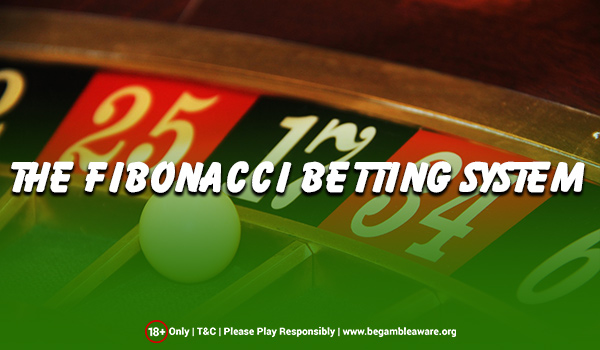# The Fibonacci Betting System – Explained

The Fibonacci Betting System comes across as a fine example of using mathematical sequences and theories to deliver superior performance in betting and gambling. The theory of negative progression system states that the stakes you have on winning bets will be much higher than the ones you have on losing bets. This is essentially the essence of increasing and decreasing wagers upon a loss and win respectively.The Fibonacci Betting System uses the Fibonacci sequence christened after Leonardo Pisano, which starts with zero, then a one and after that, every number in the sequence is the sum of the previous two numbers. The first 16 numbers of this infinite sequence are as shown below:

0, 1, 1, 2, 3, 5, 8, 13, 21, 34, 55, 89, 144, 233, 377, 610

## Usage of Fibonacci Betting System

This system can be used across a range of games like Roulette, Blackjack, Craps, Baccarat as well as Sports Betting. Its implementation involves the Fibonacci sequence but doesn’t take into account the zero at the beginning of the sequence.

Players need to decide the amount of money that would constitute one unit used in the gamble. This can be any amount as a percentage of the total amount you can gamble with. But, it is recommended that a unit comprises of 2% – 5% of your total bankroll for gambling. The 3 rules to keep in mind with the Fibonacci Betting System are:

Rule 1: The first bet in a gambling cycle should constitute one single unit. This is equal to the first number of the Fibonacci sequence, which is the number one since we have ignored the zero in the sequence for this system. As an example, if you stake £4 per unit, this bet would be for £4.

Step 2: In case of a losing bet, the units staked in the next wager must be equal to the next number in the Fibonacci sequence, which is one. If you lose again, units staked in the next bet must be the next number in the sequence, which is two. As an example, for the £4 per unit, the wager now would be £8.

Step 3: In the case of a losing bet, the units staked in the next wager must be equal to TWO numbers before the current number being used in the sequence. For example, if the winning bet was achieved after staking 21 units, the next bet must constitute 8 units. If the player wins after staking 89 units, then, the next bet must bet 34 units in the gamble.

An important point to take note while using the Fibonacci Betting System is the amount you have lost or won during every game cycle. This helps in strategising the time you should finish and go back to the start. An online betting environment enables the use of a paper or spreadsheet to track this, but in a live casino arena, you need to have a clear focus.

## Practical results of the Fibonacci Betting System

Despite being a unique strategy employed for different casino games, the Fibonacci Betting System can result in a significant downward spiral for a player if he loses continuously, as the required betting units rise very quickly in accordance with the sequence. A player running out of money remains a practical outcome before a losing streak gets reversed. The best trick to employ is to stay disciplined and cut losses if you lose a predetermined amount of money.

Rate this post

### Blog Summary

Blog Name: The Fibonacci Betting System – Explained

Posted On: 17/06/2019

Author: Robert Bowron

Rate this post